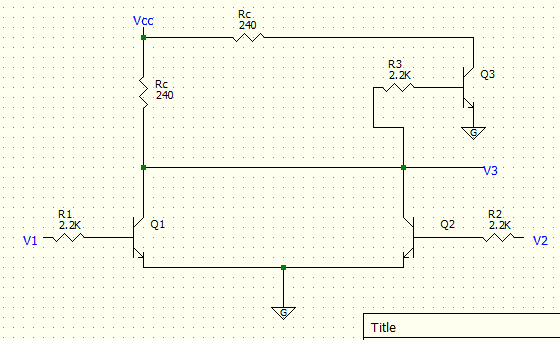# NPN Transistor Circuit Analysis

• Engineering

## Homework Statement

Determine the voltage level at V3. For each case also determine which transistors are on and saturated, on and active, and which are off. Let R1=R2=R3=2.2kΩ and let Rc=240Ω and Vcc=5V. Calculate β for each transistor as well.

We are to assume that if the transistor is on, then it is in the saturated state so Vce=0.2V. If β>20 then the transistor is in active and Vce=2V+. Also, Vbe=0.6V if the transistor is on

Case #1: V1=V2=0V
Case #2: V1=0V V2=5VIc=β*Ib
KVL, KCL

## The Attempt at a Solution

Ok, so here's what I did for the first case where both V1 and V2 are 0V. Since they are 0V, Ib=0A and therefore β=∞ for both Q1 and Q2, right? If so, then I'm basically left with the "upper" circuit containing Q3 and V3.

I then found Ib=Vcc/(240+2.2k)
Ib = 0.002A

I did the same approach for Ic=Vcc/(240)
Ic = 0.0208A

β=10.1 so Q3 is then on and in the saturated region. To find V3 I simply subtracted the voltage drop of the 240Ω resistor

V3 = 5 - (240*.002A) = 4.51V

I don't know if this is right at all since I've never done any circuit analysis with transistors before. If it's right, I'm still completely lost on how to start Case #2 where V2=5V and V1=0V. Any help is greatly appreciated.